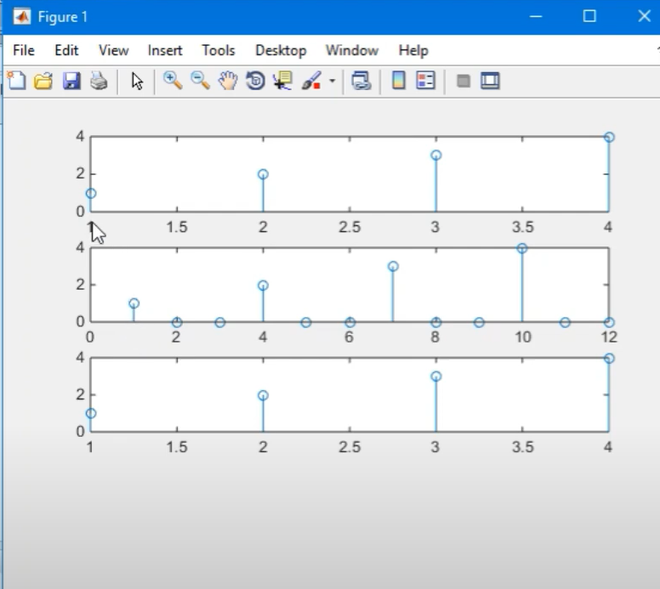# What is Upsampling in MATLAB?

• Difficulty Level : Medium
• Last Updated : 17 Dec, 2021

In this article, we will see Upsampling in MATLAB. As we know that upsampling is the process of increasing the sampling rate, i.e, increasing the number of samples. When an upsampling functions on a series of samples of a signal or other continued function, it has an estimation of the row that would have been received by sampling the signal at a higher rate. Upsampling is the method of putting zero-valued samples between actual samples to increase the sampling rate. The number of zeros between the samples is decided by the sampling factor L, (Number of zeros = L-1).

### Why Upsampling?

Often audio hardware and software offer upsampling. When analog audio is converted to digital, it needs to be filtered so that no audio enters the analog-digital converter that is higher than half the sampling rate.  The trick is to convert the digital signal to a higher sampling rate, say two or four times the original sampling rate. When done properly the audio information has not changed and thus does not contain more information, but since the signal now has a higher sampling rate, the analog filter doesn’t have to work so hard and thus causes less distortion.

Upsampling()

We have upsampled function in MATLAB that is used to increase the sampling rate of any function x[n] by sampling factor ‘L’. The basic syntax is written below.

y = upsample(a,n)

y = upsample(a,n,phase)

Here,

y = upsample(a,n) is the basic syntax of the upsampling function used in MATLAB where ‘a’ is the input signal and ‘n’ is the sampling factor. We will see an example of the function in the below code.

Suppose, we have a function x = [1 2 3 4] upsampling with a sampling factor of 3 and then downsample with the same sampling factor to get the original signal.

Example:

## Matlab

 `% MATLAB Code for upsampling ` `clc ` `clear all ` ` `  `% Original signal ` `x = [1 2 3 4]  ` ` `  `% Upsampling  ` `y = upsample(x,3) ` ` `  `% plots the data sequence ` `stem(x) ` `subplot(4,1,2) ` `stem(y) ` ` `  `% Downsampling ` `c = downsample(y,3) ` `subplot(4,1,3) ` `stem (c)`

Output:Fig 1 : Upsampling the function with the sampling factor of 3

Code Explanation:

The input signal is x has discrete values [1, 2, 3, 4 ]. The sampling factor of the upsample function is 3. Therefore the upsampled function expands the signal by adding (L-1) zeros between each sample i.e, n=3 then the number of zeros is 3-1 =2. We can see the expanded signal in the below output. Again the upsampled function is downsampled with the same scaling factor to retrieve the original signal. The ‘stem (x)’ function is used to basically plot the sequence of the data. The subplot(x,y,z) function splits the current figure into an x-by- y axis and constructs axes in the part defined by z.

My Personal Notes arrow_drop_up
Recommended Articles
Page :